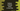# 4 Python ways to multiply the items in two lists## Python program to multiply the items in two lists:

In this post, we will learn how to create a new list by multiplying the items in two lists. This program will do index-wise multiplication i.e. the first item of the first list will be multiplied with the first item of the second list, the second item of the first list will be multiplied with the second item of the second list etc.

We can do this in different ways. Let’s take a look at them one by one:

## Method 1: By iterating the lists:

We can iterate one list and by using the iterating index, we can access the item at that index in the second list.

``````first_list = [1, 2, 3, 4, 5]
second_list = [2, 3, 4, 5, 6]
product_list = []

for i, v in enumerate(first_list):
product_list.append(first_list[i] * second_list[i])

print(f'First list: {first_list}')
print(f'Second list: {second_list}')
print(f'Product list: {product_list}')``````

Here,

• first_list and second_list are given lists of numbers.
• It iterates through the items of the first_list using a for loop and appends the product of first_list and second_list items to the product_list.
• The last three lines are printing the content of the three lists created.

If you run this program, it will print the below output:

``````First list: [1, 2, 3, 4, 5]
Second list: [2, 3, 4, 5, 6]
Product list: [2, 6, 12, 20, 30]``````

Note that it will throw one error if the length of the second list is smaller than the first list. It will throw IndexError.

``IndexError: list index out of range``

To avoid that, you can wrap the code in a try-except block:

``````first_list = [1, 2, 3, 4, 5]
second_list = [2, 3, 4, 5]
product_list = []

for i, v in enumerate(first_list):
try:
product_list.append(first_list[i] * second_list[i])
except IndexError:
print('Error..Lengths are unequal !!')

print(f'First list: {first_list}')
print(f'Second list: {second_list}')
print(f'Product list: {product_list}')``````

It will print:

``````Error..Lengths are unequal !!
First list: [1, 2, 3, 4, 5]
Second list: [2, 3, 4, 5]
Product list: [2, 6, 12, 20]``````

The length of the first list is 5 and the length of the second list is 4. The final list is created only for 4 items.

We can use list comprehension to do it quickly in just one line:

``````first_list = [1, 2, 3, 4, 5]
second_list = [2, 3, 4, 5]
product_list = [v * second_list[i]
for i, v in enumerate(first_list) if i < len(second_list)]

print(f'First list: {first_list}')
print(f'Second list: {second_list}')
print(f'Product list: {product_list}')``````

Instead of try-except block, we are checking if the value of i is less than the length of the second list or not. It will give the same output.

## Method 2: By using zip():

We can pass the lists as parameters to the zip method. This method creates a new tuple by aggregating the items of the lists. We can again use a map method to iterate over these and find the products of the items.

``````first_list = [1, 2, 3, 4, 5]
second_list = [2, 3, 4, 5]
product_list = []

for a, b in zip(first_list, second_list):
product_list.append(a*b)

print(f'First list: {first_list}')
print(f'Second list: {second_list}')
print(f'Product list: {product_list}')``````

It will work even if the lists are of different sizes.

If you run this program, it will print the below output:

``````First list: [1, 2, 3, 4, 5]
Second list: [2, 3, 4, 5]
Product list: [2, 6, 12, 20]``````

Or, we can use list comprehension:

``````first_list = [1, 2, 3, 4, 5]
second_list = [2, 3, 4, 5]
product_list = [a*b for a, b in zip(first_list, second_list)]

print(f'First list: {first_list}')
print(f'Second list: {second_list}')
print(f'Product list: {product_list}')``````

It will give the same output.

## Method 3: By using map() and list() function:

We can pass a lambda and the lists to the map function. It will create one new iterable by applying the lambda to the given lists. We can use list() to convert that iterable to a list.

The final program will be:

``````first_list = [1, 2, 3, 4, 5]
second_list = [2, 3, 4, 5]
product_list = list(map(lambda a, b: a*b, first_list, second_list))

print(f'First list: {first_list}')
print(f'Second list: {second_list}')
print(f'Product list: {product_list}')``````

It will work even if the size of the lists are different.

## Method 4: By using numpy.multiply() method:

numpy.multiply is available in NumPy library and if your project is using NumPy, you can use the multiply method to multiply two lists easily.

It is simple to use and it can handle multi-dimension lists easily. Just pass the lists as the arguments to this method and it will return the product list.

For example:

``````import numpy

first_list = [1, 2, 3, 4, 5]
second_list = [2, 3, 4, 5, 6]
product_list = numpy.multiply(first_list, second_list)

print(f'First list: {first_list}')
print(f'Second list: {second_list}')
print(f'Product list: {product_list}')``````

It will print:

``````First list: [1, 2, 3, 4, 5]
Second list: [2, 3, 4, 5, 6]
Product list: [ 2  6 12 20 30]``````

For lists with different lengths, it will throw ValueError:

``ValueError: operands could not be broadcast together with shapes (5,) (4,) ``